Next Article in Journal
Robust Force Control of Series Elastic Actuators

Actuators 2014, 3(3), 162-181; https://doi.org/10.3390/act3030162

Article
Design, Fabrication and Temperature Sensitivity Testing of a Miniature Piezoelectric-Based Sensor for Current Measurements
1
Energy Harvesting Laboratory, Department of Mechanical and Mechatronics Engineering, University of Waterloo, Waterloo, ON N2L 3G1, Canada
2
Department of Mechanical Engineering, Hamburg University of Technology, Hamburg, 21073, Germany
3
Department of Mechanical and Mechatronics Engineering, University of Waterloo, Waterloo, ON N2L 3G1, Canada
*
Author to whom correspondence should be addressed.
Received: 14 January 2014; in revised form: 3 June 2014 / Accepted: 4 June 2014 / Published: 9 July 2014

Abstract

:
Grid capacity, reliability, and efficient distribution of power have been major challenges for traditional power grids in the past few years. Reliable and efficient distribution within these power grids will continue to depend on the development of lighter and more efficient sensing units with lower costs in order to measure current and detect failures across the grid. The objective of this paper is to present the development of a miniature piezoelectric-based sensor for AC current measurements in single conductors, which are used in power transmission lines. Additionally presented in this paper are the thermal testing results for the sensor to assess its robustness for various operating temperatures.
Keywords:
AC current sensor; piezoelectric sensor; non-intrusive sensor; PZT; bimorph; force on a magnet; AC magnetic field; smart grid

1. Introduction

The need to improve the reliability and efficiency of electrical distribution has prompted an increased interest in developing new technologies and devices for the smart grid. The development of the smart grid requires the monitoring and active management of electrical demand . An array of wireless current sensors distributed on a network of transmission lines is envisioned to achieve the monitoring aspect. However, due to the immense size of distribution networks, low cost and easily maintainable sensors are required to allow this large-scale deployment. Furthermore, with the necessary retrofitting of existing transmission lines, a non-intrusive sensor that can be installed without service disruptions would be beneficial.
Some examples of existing non-contact current sensors are Hall sensors, Rogowski coils and magnetoresistive sensors . However, size, costs and external power requirements are some limitations of these technologies . These non-contact sensors operate by interacting with the magnetic field induced by the current in the wire to be measured. Another method of interacting with this magnetic field is to place a permanent magnet within close proximity. The force from the reacting magnetic fields can then be sensed by attaching the magnet to the tip of a piezoelectric cantilever. Due to the characteristics of a piezoelectric material (PZT), the sensor would produce a voltage that can be correlated to the current in the wire [2,3,4,5,6,7,8]. Existing designs that use this principle pertain to sensors that are used mainly for circuit breaker panels and dual cable measurements [3,6,9,10]. Similar techniques with cantilevers have also had much success in multiple other sensing applications, such as biological, chemical and physical, by measuring changes in the resonance response or deflection of the cantilever .
This paper presents the theoretical development, design, fabrication and experimental results of a miniature non-intrusive AC current sensor. This sensor uses the principle described above and allows measurements of multiple harmonics of currents in single conductors primarily used in power transmission lines. The design and magnet orientation proposed in this paper particularly allows efficient interactions with the magnetic field of a single conductor transmission line which helps maximize the sensor sensitivity for this application. Furthermore, we assess thermal robustness of the sensor by examining the effects of the temperature variations on the sensor sensitivity.

2. Theoretical Model

The sensor consists of a brass substrate beam with two piezoelectric layers and a magnet as shown in Figure 1. In the proximity of a wire carrying AC current, the alternating magnetic flux around the wire produces an alternating force on the magnet which results in vibrations of the substrate and piezoelectric layers . The resulting AC voltage can then be measured and the sensor can be calibrated for current measurements.
Figure 1. Schematic of the miniature AC current sensor. (a) Side view; (b) Front view.
Figure 1. Schematic of the miniature AC current sensor. (a) Side view; (b) Front view.
Presented in this section are the electromagnetic force equation derivations and dynamic modelling of the substrate beam with piezoelectric layers. Two sensor orientations are suggested and compared for the case of single conductor measurements. For clarification, Figure 2 shows the magnetic field schematics of single and double conductors. The double conductors are common in electric appliances, which contain the ground (return) wire and are not the intended application for the sensor described in this paper.
Figure 2. Magnetic fields of single and double conductors carrying current. (a) Single conductor; (b) Double conductor.
Figure 2. Magnetic fields of single and double conductors carrying current. (a) Single conductor; (b) Double conductor.

2.1. Electromagnetic Force Modelling

Presented in this section is the derivation for the electromagnetic force on the sensor tip magnet. The interaction between the magnet and the transmission wire can be better understood using Equations (1) and (2). Equation (1) gives the force on a current carrying wire element placed in an external magnetic field .
dF = I (dl × Bmag)
Here, I is the current in the wire, dl is the differential wire element vector (directed along the direction of current flow) and Bmag is the magnetic flux density of the magnetic field produced by the magnet. This force on the wire is equal and opposite to the force on the magnet element. Equation (2) gives the force on a magnetic dipole element placed in an external magnetic field by the wire as illustrated in Figure 3 .
dF = (dmBwire)
Here, dm is the magnetic moment of the magnetic dipole element and Bwire is the time varying magnetic flux density of the magnetic field produced by the wire.
Figure 3. Magnetic dipole element placed in the magnetic field of a current carrying wire.
Figure 3. Magnetic dipole element placed in the magnetic field of a current carrying wire.
The magnetic moment of the dipole element shown in Figure 4 can be written aswhere dmx, dmy, and dmz are the x, y and z components for any arbitrary orientation of the dipole.
Figure 4. Magnetic dipole element and the magnetic moment.
Figure 4. Magnetic dipole element and the magnetic moment.
In addition, the magnitude of the dipole moment can be expressed as
dm = Br (dV / μ)
where Br is residual magnetic flux density or remanence, dV is the differential volume of the element, and μ is the permeability of air. From Equation (1) it can be seen that, to maximize the force on a current carrying element, it is necessary to maximize the cross product of the external magnetic flux density and the current unit vector. This can be achieved through two different orientations of the magnet. Figure 5 shows the two orientations that allow the magnetic field lines of a single magnet to be approximately perpendicular to the wire. The dashed lines represent the magnetic field lines of the wire and the solid lines represent the magnetic field lines of the magnet. In orientation “a”, the magnet is placed such that its magnetic axis is tangent to the lines that represent the magnetic field of the wire. In orientation “b”, the magnet axis is perpendicular to these lines. Both orientations result in a net force on the wire that is perpendicular to the axis of the magnet. Once again, note that the force on the wire is equal and opposite of the force on the magnet. Intermediate orientations lead to both a reduction in efficiency of the magnetic field interactions, and asymmetric forces, which are undesirable for the sensor design.
Figure 5. Magnetic fields for “a” and “b” magnet orientations.
Figure 5. Magnetic fields for “a” and “b” magnet orientations.
Figure 6. Coordinate system for a single conductor.
Figure 6. Coordinate system for a single conductor.
For simplicity, Equation (2) is used instead of Equation (1) to obtain the expressions for the total force on a tip magnet as described below. Figure 6 shows the coordinate system used for obtaining these expressions. In this figure, r is the position vector of any point (y,z) around the wire (r =). The wire is placed along the x axis, therefore:Here Ia is the current amplitude, v is the frequency and t is time.
Using Ampere’s law ,the magnetic field around an infinitely long current carrying wire can be expressed as:Using the coordinate system show in Figure 6, Equation (7) simplifies to: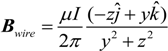By substituting Equations (3) and (8) in Equation (2), the force on the differential volume of the magnetic element can be found as:For orientation “a”, the j components of the force cancel out due to symmetry. In addition, the magnetic dipole moment along the i and k directions is zero. Thus, for this orientation, the following expression for the force on a differential element volume is obtained using Equations (4) and (9):Similarly, the following expression is obtained for orientation “b”:The total force on the magnet can then be found by integration over the magnet volume. Figure 7 and Figure 8 present the theoretical force per unit current and unit residual flux density for both orientations “a” and “b” for various distances between the wire and the magnet. The results are obtained for both cuboid and cylindrical magnets. Three different magnet lengths of 0.5 cm, 1 cm, and 1.5 cm with a 1 cm2 cross section are used for both geometries. As illustrated in these figures, the optimal orientation (“a” or “b”) depends on the magnet length. For both cuboid and cylindrical geometries, orientation “a” is shown to produce greater magnetic force values if the length of the magnet is larger than the width (diameter). For lengths equal to the width (diameter) both configurations give similar results.
For the prototype, a cylindrical magnet and orientation “a” were chosen. Orientation “b” is not the most suitable option for a single wire measurement because the force along the magnet varies along its length due to the varying distances of the magnetic elements from the wire. This will result in additional torsional vibrations, which are not desired for the sensor measurement calibration. Additionally, a cylindrical magnet was selected as it allows for a smaller contact area with the beam. This geometry simplifies the dynamic modelling of the sensor since it allows modelling the magnet as a tip mass.
Figure 7. Theoretical force per unit current and unit residual flux density for cuboid magnets.
Figure 7. Theoretical force per unit current and unit residual flux density for cuboid magnets.
Figure 8. Theoretical force per unit current and unit residual flux density for cylindrical magnets.
Figure 8. Theoretical force per unit current and unit residual flux density for cylindrical magnets.

2.2. Piezoelectric Modelling

The schematic of the proposed model of the sensor is shown in Figure 9. This sensor configuration allows a more effective forcing mechanism for the sensor compared to Reference  due to the chosen orientation of the magnet with respect to the sensor substrate layer.
A mathematical model is required to predict the sensor output and its dynamic behaviour. The sensor must be designed for the frequency range of interest in such a way that its natural frequency is far from the frequencies of operation. This results in a fairly constant frequency response function (FRF) for the frequency range of interest, which aids in obtaining a non-variable calibration ratio. Additionally, this results in relatively small cantilever deflections for these frequencies as they are far from resonance. Therefore, the deflections are also assumed to have no significant influence on the electromagnetic forces on the tip magnet and this force is assumed to have a harmonic form.
Figure 9. Schematic of the cantilevered beam sensor.
Figure 9. Schematic of the cantilevered beam sensor.
In order to obtain the natural frequency of the sensor, the governing partial differential equation (PDE) for base excitations applied to the clamped end are considered. The theoretical frequency response function for the sensor output voltage to base excitation is later validated through a shaker harmonic testing. The PDE for the sensor depicted in Figure 9 may be found as follows: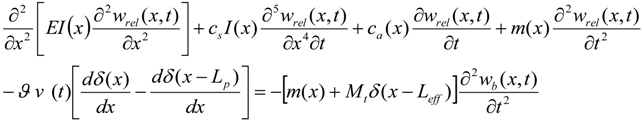The transverse deflection of the beam relative to the base input excitation at position x and time t is wrel (x,t), while the base excitation is denoted by wb (x,t). Note that the total deflection can be found as wtotal (t) = wb (t) + wrel (t). The terms csI and ca are the strain rate damping and air damping terms respectively. Air damping is assumed to be negligible in this analysis. The strain rate damping, known as Kelvin-Voight damping, is later incorporated in the modal coordinates through model damping ratios obtained from the experiments . Leff is the effective length of the beam which is measured from the clamped end to the center of the magnet and Mt is the total tip mass which includes the magnet, the tip of the beam and the epoxy bonding the two. Finally, v(t) and ϑ are the voltage and the electromechanical coupling term for the piezoelectric layers in a parallel configuration respectively. The electromechanical coupling term ϑ is given by:Here,is a piezoelectric coupling constant, tp and ts are the thicknesses of the piezoelectric (one layer) and substrate material, respectively, w is the width of the beam and npzt is the distance from the neutral axis of the substrate to the neutral axis of the piezoelectric layer. Equation (12) as a whole is very similar to the expression found in , however, due to the discontinuity in the piezoelectric material, care has been taken to modify the mass per unit length term m(x) and the bending stiffness term EI(x) .

2.2.1. Mode Shape Functions

Using mode summations, the response of the system can be described as a series of eigenfunctions (mode shapes) as is commonly done using the separation of variables:Here, ϕr (x) is the mass normalized eigenfunction for an undamped vibration, and ηr (t) is the modal mechanical coordinate expression for the rth vibration mode. However, due to the discontinuity of the piezoelectric layer in the beam, the solution to the spatial ODE is segmented in piecewise sections :
For piezoelectric-substrate (Section 1): 0 < x < Lp
ϕr,1(x) = Ar,1 cos(βr,1x) + Br,1 cosh(βr,1x) + Cr,1 sin(βr,1x) + Dr,1 sinh(βr,1x)
For substrate only (Section 2): Lp < x < Leff
ϕr,2(x) = Ar,2 cos(βr,2x) + Br,2 cosh(βr,2x) + Cr,2 sin(βr,2x) + Dr,2 sinh(βr,2x)
Here constants βr,1 and βr,2 are mode shape parameters for each of the two sections. The relation between βr,1 and βr,2 may be found as :where m1 and m2 are the mass per unit lengths for each of the two sections. Also, (EI)1 and (EI)2 are the bending stiffness of the two sections.

2.2.2. Boundary Conditions

The boundary conditions and continuity equations that describe the system shown in Figure 9 are presented in this section. Equations (18) and (19) are the boundary conditions at the clamped end (x = 0):
φr,1(0) = 0Equations (20) to (23) are the continuity conditions between the two segments of the beam:
φr,1(Lp) = φr,2(Lp)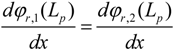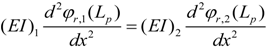Equations (24) and (25) are the boundary conditions at the free end of the beam (x = Leff):Here, It and Mt are the mass moment of inertia and mass of the tip mass. The stated boundary and continuity conditions can be described in the matrix form as follows:
P.Q = 0
where Q is a vector of the mode shape coefficients and P is the multiplier matrix:
Q = [Ar,1,Br,1, Cr,1,Dr,1,Ar,2,Br,2,Cr,2,Dr,2]T
For a non-trivial solution, the determinant of P has to vanish. Using this method, the natural frequencies (short-circuit condition) of the system can then be found as:2.2.3. Governing Equations of Motion

The equations for the modal coordinates can then be determined using Equations (12) and (14) and applying the orthogonality condition as: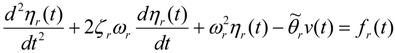where the modal electromechanical coupling term is:The modal mechanical forcing function for base acceleration is described as:and the modal mechanical damping ratio ζr is found using experimental results through the half power method.

2.2.4. Electrical Circuit Equation

The coupled electrical circuit equation for the bimorph connection in parallel can be derived using Kirchhoff’s law in which the piezoelectric layers are modelled as two current sources in parallel with internal capacitances as shown in Figure 10 . The large internal resistance of the measurement unit results in an open circuit condition.
Figure 10. Piezoelectric sensor circuit representation (parallel circuit connection).
Figure 10. Piezoelectric sensor circuit representation (parallel circuit connection).
Using Kirchhoff’s law the following equation is formed.Here, Rl is the load resistance (measurement unit), andis the effective capacitance of both piezoelectric layers. This equation represents the coupled electrical circuit equation used to determine the voltage response of the sensor due to base excitations.

2.2.5. Frequency Response Function and Forcing Functions

Assuming harmonic functions, i.e., ηr(t) = Hrejωt and v(t) = Vejωt, the steady state modal mechanical response of the beam and steady state voltage response across the resistive load, Equations (29) and (32) become:By substitution of Equations (33) and (34), one can obtain the open circuit natural frequency and steady-state voltage response as :The voltage output to base acceleration FRF can then be found as:where Fr = −σrω2Wo defines the base acceleration forcing function, σr defines the forcing function as:and W0 is the base displacement amplitude. The FRF found using this method can then be validated through testing by mounting the sensor on a shaker that provides base acceleration in order to produce the voltage output in the piezoelectric layers. The analytical and experimental test results are compared and discussed later in Section 3 of this paper.
The second part of this modelling focuses on obtaining the sensor sensitivity which is defined as the sensor output voltage per input current passing through a wire in the proximity of the sensor. As discussed previously, the input current results in an electromagnetic force on the tip magnet. This force can be obtained by integrating Equation (10) over the volume of the cylindrical magnet shown in Figure 9 as follows:Here, h is the distance from the center of the wire to the closest point of the magnet, the length of the magnet L is measured along the magnetic axis and R is the radius of the magnet. This relation is then used to obtain the output voltage for the sensor when placed at the proximity of a current carrying wire.

2.2.6.1. Voltage Loss

If a voltage measurement unit with finite inner resistance is connected to the contacts of the piezoelectric sensor, a current Iloss will flow and, thus, the charge displacement on the piezoelectric electrodes will change. Figure 11 shows this equivalent circuit and the current loss schematic.
Figure 11. Equivalent circuit and the current leakage.
Figure 11. Equivalent circuit and the current leakage.
This change in charge displacement will result in a voltage loss across the piezoelectric layers, which is not desirable for a sensing application. Using Ohm’s law and the constitutive equations for a piezoelectric bimorph, the following equation may be found for the relative voltage-loss in a quarter period of the oscillating voltage across the piezoelectric layers.Here, v0 is the amplitude of the output voltage, Tperiod is the period of the sinusoidal function, Ri is the inner resistance of the voltage measurement unit, which is assumed to be 100 times the impedance of the sensor (see Section 2.2.6.2), andis the dielectric permittivity at constant strain. A value of approximately 2.36% was obtained for this ratio for the sensor after parameter optimization, which is acceptable for the design criteria. In addition, gravity effects, temperature expansion or an offset of the input signal may all produce a static offset for the sensor measurements. However, this is not a concern for an AC current sensor since the offset will be in the form of a static signal and will decay after a short period of time.

2.2.6.2. Sensor Impedance

As shown by Staines et al. , and also demonstrated in Equations (39) and (40), the voltage loss through the sensor is inversely proportional to the capacitance of the sensor. Note that the sensor capacitance is also inversely proportional to its impedance. Hence, generally, the sensor must be designed in a way that its impedance is low (high capacitance) compared to the inner resistance of the measurement device in order to reduce the measurement noise level. A ratio of 100 was considered between the impedance of the sensor and the voltage measurement unit to be used during the sensor’s actual operation. On the other hand, the sensor must be designed to guarantee an operation mode close to an open circuit condition, which ultimately requires a large impedance. The sensor capacitance under unstrained condition is defined as: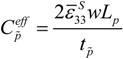Here,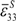is the dielectric permittivity of the piezoelectric material. The factor of two appears because the sensor is configured in parallel mode. The sensor impedance was found to be about 55 kΩ.

2.2.6.3. Electromagnetic Loss

The substrate material is a nonmagnetic material and is used in commercially available bimorph sensors and actuators. In addition, the induced eddy currents due to vibration of the substrate and the variable magnetic field are assumed negligible in this research. These eddy currents will result in additional damping and power loss by the substrate that can be approximated by :where Ploss is the total power dissipation, Bp is the peak flux density, f is the frequency at which magnetic flux density changes, and ρs is the resistivity of the substrate. Therefore, due to the relatively high resistivity of the brass substrate and its small thickness, the power loss due to the eddy current effects is ignored.

2.2.6.4. Final Design Parameters

The design specifications and constraints considered for this sensor are shown below in Table 1.
Table 1. Design specifications and constraints considered for the sensor design.
Table 1. Design specifications and constraints considered for the sensor design.
SpecificationValue/Range
Sensor operating current range 10 A–200 A
Sensor accuracy within 1% @ 10–100 A, 4% @ 100–200 A
Operating temperature −40 °C to 80 °C
Output voltage for 10,000 A ±1.75 V
Sensor impedance 55 kΩ
The internal impedance of the sensor was chosen to be small compared to the voltmeter circuit inner impedance in order to reduce the measurement noise level as explained previously. Based on the design constraints, the values shown in Table 2 were obtained and selected for the sensor dimensions and other parameters. A D66SH (K&J Magnetics) magnet and the PZT-5A piezoelectric material were selected as they were the most suitable for the wide range of design temperatures. In particular, PZT-5A has a high sensitivity and very good temperature stability over the operating range of temperatures and is commonly used for commercially available sensors and actuators .
Table 2. Sensor Parameters.
Table 2. Sensor Parameters.
PropertyValue/Type
Substrate materialBrass 260 (McMaster Carr)
Ls26 mm
ts1.55 mm
w14.45 mm
Leff20.5 mm
Piezoelectric materialPZT-5A4E (Piezo Systems, Inc.)
ξ0.75
Lpξ · Leff (mm)
tp0.127 mm (each layer)
Magnet D66SH (K&J Magnetics)
Rl1 MΩ (Measurement Device Resistance)

3. Testing

The fabricated sensor, shown in Figure 12, consists of a brass alloy 260 substrate, a neodymium magnet (K&J Magnetics D66SH) and two laser-cut piezoelectric layers of PZT-5A (Piezo System T105-A4E-602). The sensor was assembled using two grades of epoxy. One was an aircraft grade epoxy (Loctite Hysol E-120HP), which was used to attach the magnet and the piezoelectric material to the substrate. The other was an electrically conductive epoxy (MG Chemical 8331), which was used to create electrically conductive bonds between the piezoelectric material and the substrate, and between the piezoelectric material and the measurement wires. As shown in Figure 12, the sensor was mounted onto a current carrying wire (single conductor) and the voltage generated by the sensor was measured and recorded.
Figure 12. Current sensor prototype testing.
Figure 12. Current sensor prototype testing.

3.1. Sensor Sensitivity

The facilities in an Ottawa Hydro test lab were used for the sensor sensitivity testing. A variable voltage supply was placed under a resistance load and the current was measured. Sensor peak voltage readings were obtained at various distances from a current carrying wire. The test results shown in Figure 13 demonstrate the linearity of the response for currents up to 150 A (RMS), which aids with the sensor calibration. Figure 14 shows the theoretical and measured sensor sensitivities for varying distances. As expected, the sensor sensitivity increased when the sensor was mounted closer to the wire. As shown in this figure, the theoretical and experimental results are in very good agreement.
Figure 13. Experimental sensor output voltage for various currents.
Figure 13. Experimental sensor output voltage for various currents.
Figure 14. Theoretical and experimental sensor sensitivity.
Figure 14. Theoretical and experimental sensor sensitivity.

3.2. Thermal Sensitivity

One major requirement for using this sensor in various environments is to ensure that the sensor design is thermally robust. To examine the temperature dependence of the sensor sensitivity, the sensor was placed inside a CSZ MicroClimate Benchtop Test Chamber for sensitivity testing. A wire was run through the chamber and loaded with a 1500 W portable space heater. For comparison purposes, a Fluke i400s current clamp (15 cm × 7 cm × 3 cm) was used to measure the actual current in the wire.
Figure 15. Experimental sensor sensitivity for various temperatures and sensor-wire distances.
Figure 15. Experimental sensor sensitivity for various temperatures and sensor-wire distances.
Figure 15 presents the sensitivity data for the sensor at various temperatures and distances from the wire. These sensitivity values were found based on multiple current measurements. For each temperature setting, the thermal chamber was left for an hour in order to stabilize its temperature before the sensor measurements were taken. As shown in Figure 15, the smaller the distance between the sensor and the wire, the larger the temperature effects on the sensor readings. Therefore, there is a trade-off between the distance between the sensor and the wire and the accuracy of the current sensor measurements. It is not ideal to locate the sensor at large distances from the wire due to low sensor sensitivity. On the other hand, small distances increase the dependence of the sensor readings on the ambient temperatures. The results show that a 30 mm distance between the sensor and the wire exhibits a fairly robust thermal behaviour for the sensor readings.

3.3. Natural Frequency and FRF Testing

As stated previously in this paper, in order to be able to effectively calibrate the sensor over a wide range of harmonics, the fundamental natural frequency of the sensor should be sufficiently larger than the highest frequency of interest. A natural frequency of about 800 Hz was chosen for this design. The natural frequency of the sensor was measured through modal testing using a Model Shop Model 2075E dual-purpose electromagnetic shaker as shown in Figure 16. To ensure no interference between the sensor tip magnet and the magnetic core of the shaker, an aluminum spacer was mounted on the shaker to provide base excitations to the sensor. Sine sweep testing with a constant acceleration (0.42 g) was performed to obtain the FRF of the sensor due to shaker excitations. An LMS SCADAS Mobile SCM05 data acquisition system was used for the FRF measurements. The natural frequency obtained using this test was 781 Hz.
Figure 16. Sensor modal testing setup. (a) Overview of setup; (b) Close-up of sensor mounted on the electromechanical shaker.
Figure 16. Sensor modal testing setup. (a) Overview of setup; (b) Close-up of sensor mounted on the electromechanical shaker.
The theoretical fundamental natural frequency of the system can be determined for both short and open circuit conditions using the model presented in the previous section of the paper. Based on the theoretical model, fundamental natural frequency values of 794.85 Hz and 815 Hz were predicted for the short circuit condition (Rl = 0) and the open circuit condition (Rl = 1 MΩ) respectively. This constitutes an overestimation error of less than 5% between the model and experimental open circuit fundamental natural frequencies. Additionally, the eddy current effects in the substrate, PZT layers, and the magnet are assumed to be negligible and are not accounted for in this model.
Figure 17. Theoretical and experimental FRF (open circuit condition).
Figure 17. Theoretical and experimental FRF (open circuit condition).

3.4. Current Harmonic Testing

The PZT sensor and the Fluke i400s current clamp were used to measure the current running through a wire loaded by a 1500 W space heater. The piezoelectric sensor voltage output was calibrated using a constant ratio for the range of frequencies to find the current measurements for this sensor based on the current clamp readings. The results are shown and compared in Figure 18. It was observed from the tests that the voltage from the PZT sensor was proportional to the current in the wire for the range of frequencies of interest for any given distance between the sensor and the wire. As presented in this figure, the results show very good agreement between the current clamp and the piezoelectric based sensor for the designed range of frequencies, i.e., the first five harmonics (0–300 Hz). Additionally demonstrated in this figure is the diminished accuracy of the PZT sensor measurements for frequencies in the vicinity of the fundamental natural frequency of the sensor. As explained previously, in order to obtain a constant output voltage to current ratio for a range of frequencies, the highest frequency of interest should be far below the fundamental frequency. For this design, a value of 2.5 was found for the ratio between the fundamental frequency and the highest frequency of interest to provide accurate readings for the first 5 current harmonics.
Figure 18. Experimental current measurements of the sensor prototype and a Fluke i400s.
Figure 18. Experimental current measurements of the sensor prototype and a Fluke i400s.

4. Conclusions

A miniature piezoelectric material based sensor was designed to measure current in single conductors used in power transmission lines. The model predictions for the natural frequency and the sensor sensitivity are in strong agreement with the experimental test results. Testing was also performed in a thermal chamber to assess thermal robustness of the fabricated sensor and to evaluate its thermal sensitivity. The sensor shows excellent accuracy compared to a conventional current measurement device while being about 100 times smaller in volume.

Acknowledgment

The authors graciously acknowledge the NSERC funding agency in Canada for supporting this research under the NSERC-EGP 428973-2011 and partially under NSERC-DG 371472-2009.

Author Contributions

Steven Lao was responsible for the fabrication of the prototype, the experimental testing and data analysis. Shamsheer Chauhan was responsible for the magnetic force modelling and proposing the magnet configuration. Thorben Schröder was responsible for the initial dynamic modelling and design optimization of the prototype. Tim Pollock and Shamsheer Chauhan were responsible for developing the revised dynamic model presented in this paper. In Sik Cho was responsible for the fabrication of the prototype. Dr. Armaghan Salehian developed the major ideas for this research and supervised the project.

Conflicts of Interest

The authors declare no conflict of interest.

References

1. NIST Framework and Roadmap for Smart Grid Interoperability Standards, Release 2.0, National Institute for Standards and Technology (NIST): Gaithersburg, MD, USA, 2012.
2. Leland, E.S.; White, R.M.; Wright, P.K. Energy scavenging power sources for household electrical monitoring. In Proceedings of the PowerMEMS 2006, Berkeley, CA, USA, 29 November–1 December 2006; pp. 165–168.
3. Leland, E.S.; White, R.M.; Wright, P.K. Design of a MEMS passive, proximity-based AC electric current sensor for residential and commercial loads. In Proceedings of the PowerMEMS 2007, Freiburg, Germany, 28–29 November 2007; pp. 77–80.
4. Roundy, S.; Wright, P.K. A piezoelectric vibration based generator for wireless electronics. Smart Mater. Struct. 2004, 13, 1131–1142. [Google Scholar]
5. Leland, E.S.; Wright, P.K.; White, R.M. A MEMS AC current sensor for residential and commercial electricity end-use monitoring. J. Micromech. Microeng. 2009, 19, 094018. [Google Scholar] [CrossRef]
6. Leland, E.S.; Sherman, C.T.; Minor, P.; Wright, P.K.; White, R.M. A self-powered MEMS sensor for AC electric current. In Proceedings of the PowerMEMS 2009, Washington, DC, USA, 1–4 December 2009; pp. 53–56.
7. Leland, E.S.; Sherman, C.T.; Minor, P.; White, R.M.; Wright, P.K. A new MEMS sensor for AC electric current. In Proceedings of the IEEE Sensors 2010 Conference, Kona, HI, USA, 1–4 November 2010; pp. 1177–1182.
8. Paprotny, I.; Xu, Q.; Chan, W.W.; White, R.M.; Wright, P.K. Electromechanical Scavenging from current-carrying conductors. IEEE Sens. J. 2013, 13, 190–201. [Google Scholar]
9. Xu, Q.R.; Paprotny, I.; Seidel, M.; White, R.M.; Wright, P.K. Stick-on piezoelectromagnetic AC current monitoring of circuit breaker panels. IEEE Sens. J. 2013, 13, 1055–1064. [Google Scholar] [CrossRef]
10. Paprotny, I.; Leland, E.; Sherman, C.; White, R.M.; Wright, P.K. Self powered MEMS sensor module for measuring electrical quantities in residential, commercial, distribution and transmission power systems. In the Proceedings of the 2010 IEEE Energy Conversion Congress and Exposition (ECCE), Atlanta, GA, USA, 12–16 September 2010; pp. 4159–4164.
11. Thundat, T.; Oden, P.I.; Warmack, R.J. Microcantilever Sensors. Microsc. Thermophys. Eng. 1997, 1, 185–199. [Google Scholar] [CrossRef]
12. Ida, N.; Bastos, J.P.A. Electromagnetics and Calculations of Fields; Springer-Verlag New York Inc.: New York, NY, USA, 1992; pp. 175–182. [Google Scholar]
13. Boyer, T.H. The force on a magnetic dipole. Am. J. Phys. 1998, 56, 688–692. [Google Scholar] [CrossRef]
14. Erturk, A.; Inman, D.J. Piezoelectric Energy Harvesting; John Wiley & Sons, Ltd.: Chichester, UK, 2011; pp. 23–73. [Google Scholar]
15. Bashash, S.; Salehi-Khojinl, A.; Jalili, N. Forced vibration analysis of flexible Euler-Bernoulli beams with geometrical discontinuities. In Proceedings of the 2008 American Control Conference, Seattle, WA, USA, 11–13 June 2008; pp. 4029–4034.
16. Staines, G.; Hofmann, H.; Dommer, J.; Altgilbers, L.; Tkach, Y. Compact Piezo-Based High Voltage Generator - Part I: Quasi-Static Measurements. Electromagn. Phenom. 2003, 3, 373–383. [Google Scholar]
17. Fiorillo, F. Characterization and Measurements of Magnetic Materials; Elsevier Academic Press: San Diego, CA, USA, 2004. [Google Scholar]
18. Hooker, M.W. Properties of PZT-based Piezoelectric ceramics between −150 and 250 °C, National Aeronautics and Space Administration. Langley Research Center: Hampton, VA, USA, 1998. [Google Scholar]